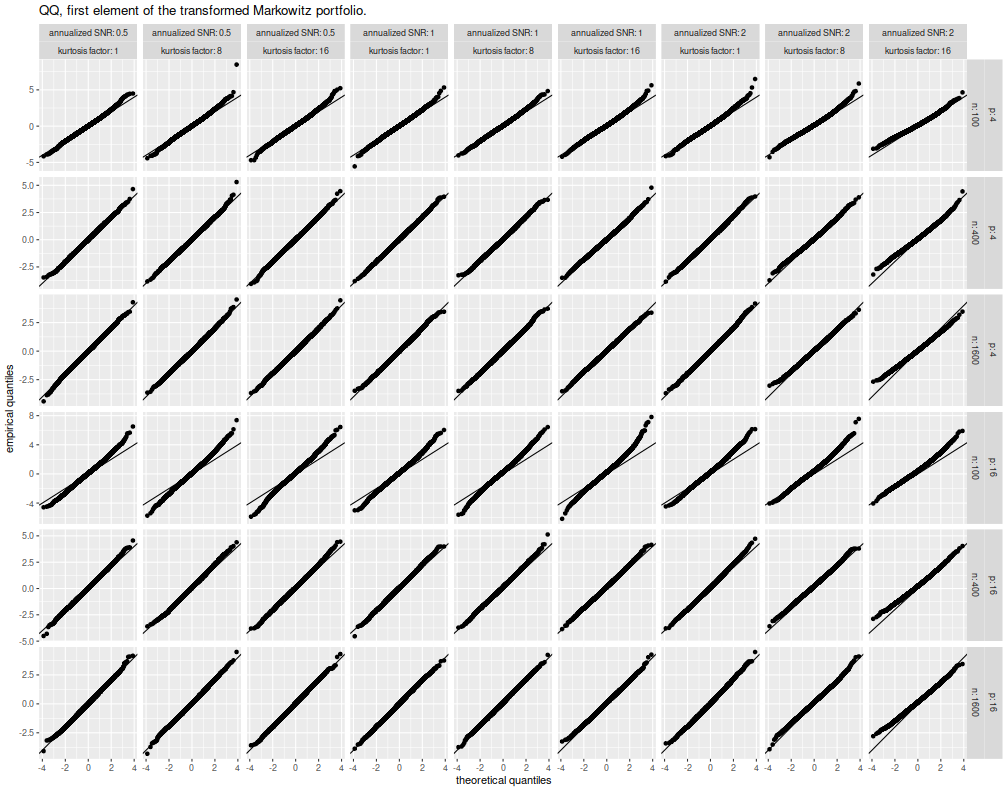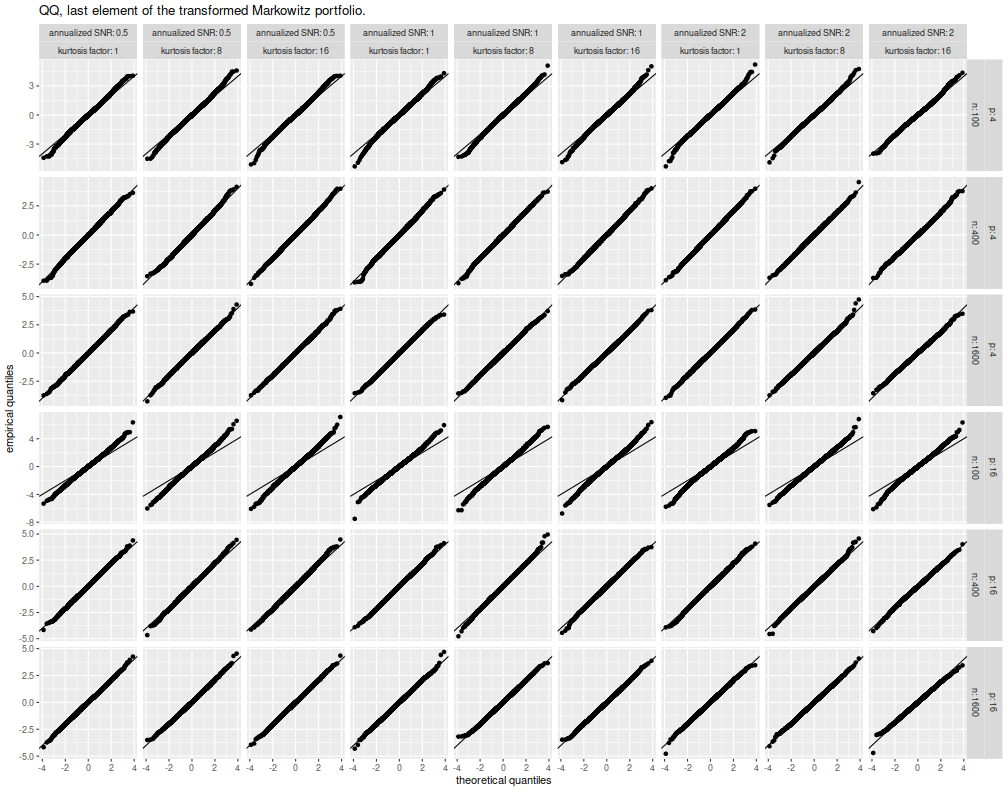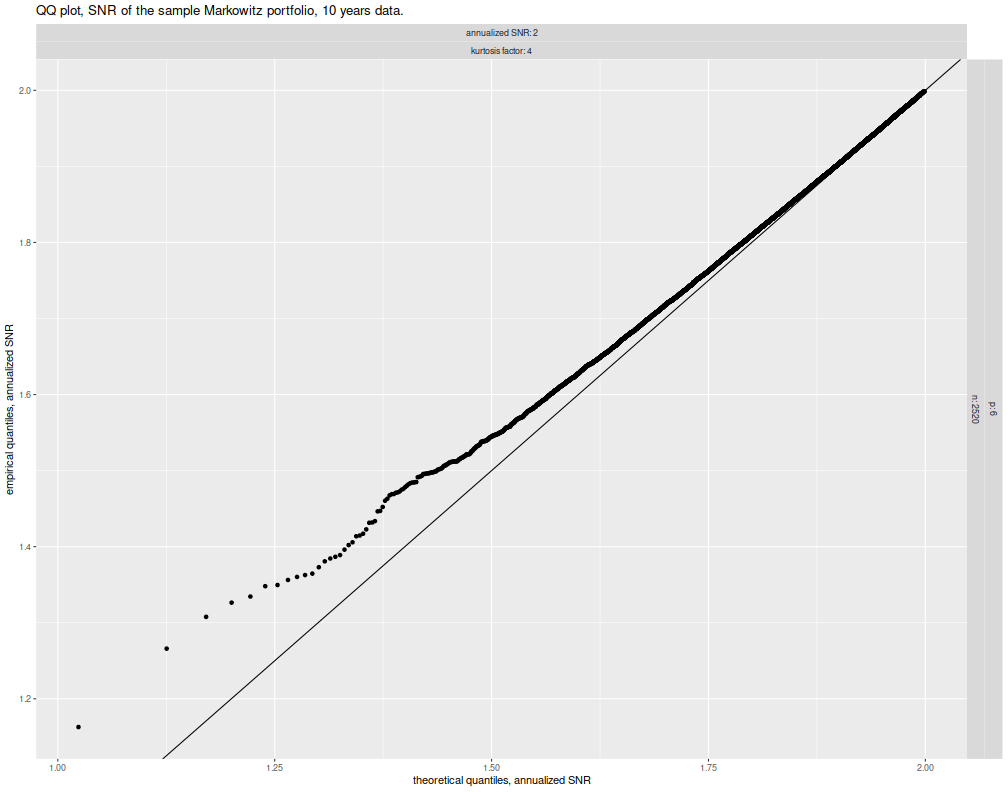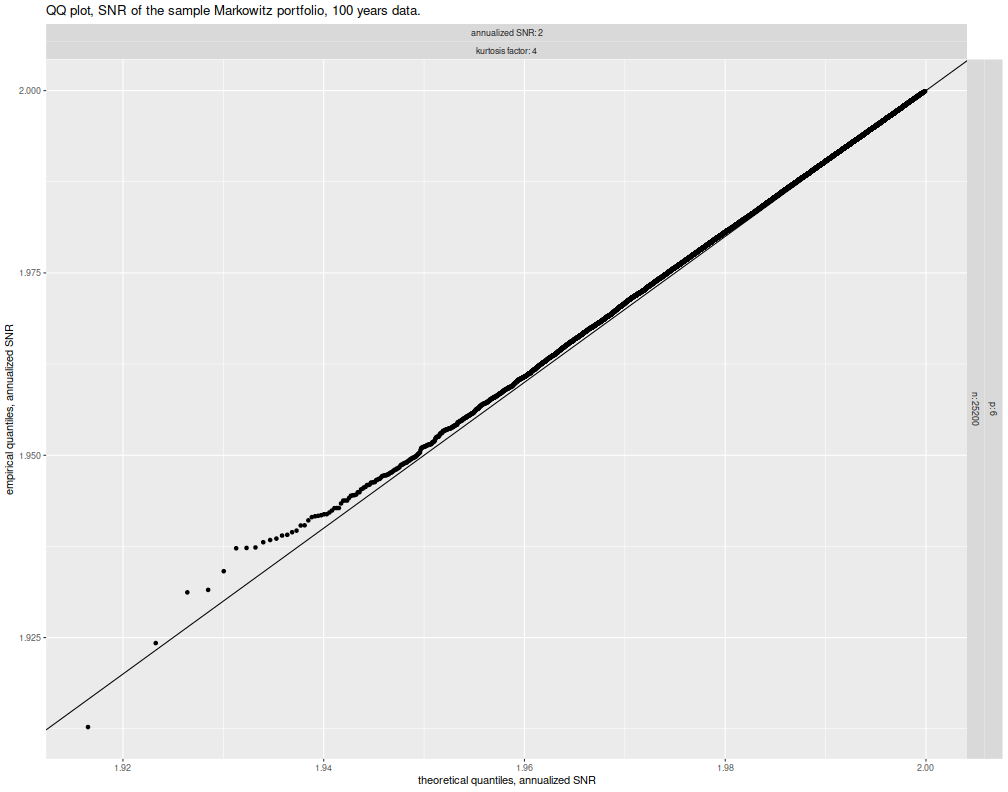In a previous blog post, I looked at asymptotic confidence intervals for the Signal to Noise ratio of the (sample) Markowitz portfolio, finding them to be deficient. (Perhaps they are useful if one has hundreds of thousands of days of data, but are otherwise awful.) Those confidence intervals came from revision four of my paper on the Asymptotic distribution of the Markowitz Portfolio. In that same update, I also describe, albeit in an obfuscated form, the asymptotic distribution of the sample Markowitz portfolio for elliptical returns. Here I check that finding empirically.

Suppose you observe a $$p$$ vector of returns drawn from an elliptical distribution with mean $$\mu$$, covariance $$\Sigma$$ and 'kurtosis factor', $$\kappa$$. Three times the kurtosis factor is the kurtosis of marginals under this assumed model. It takes value $$1$$ for a multivariate normal. This model of returns is slightly more realistic than multivariate normal, but does not allow for skewness of asset returns, which seems unrealistic.

Nonetheless, let $$\hat{\nu}$$ be the Markowitz portfolio built on a sample of $$n$$ days of independent returns:

$$\hat{\nu} = \hat{\Sigma}^{-1} \hat{\mu},$$

where $$\hat{\mu}, \hat{\Sigma}$$ are the regular 'vanilla' estimates of mean and covariance. The vector $$\hat{\nu}$$ is, in a sense, over-corrected, and we need to cancel out a square root of $$\Sigma$$ (the population value). So we will consider the distribution of $$Q \Sigma^{\top/2} \hat{\nu}$$, where $$\Sigma^{\top/2}$$ is the upper triangular Cholesky factor of $$\Sigma$$, and where $$Q$$ is an orthogonal matrix ($$Q Q^{\top} = I$$), and where $$Q$$ rotates $$\Sigma^{-1/2}\mu$$ onto $$e_1$$, the first basis vector:

$$Q \Sigma^{-1/2}\mu = \zeta e_1,$$

where $$\zeta$$ is the Signal to Noise ratio of the population Markowitz portfolio: $$\zeta = \sqrt{\mu^{\top}\Sigma^{-1}\mu} = \left\Vert \Sigma^{-1/2}\mu \right\Vert.$$ A very recent paper on arxiv calls $$\Sigma^{-1/2}\mu$$ the 'Generalized Information Ratio', and I think it may be productive to analyze this quantity.

Back to our problem, as $$n$$ gets large, we expect $$\hat{\Sigma}$$ to approach $$\Sigma$$ in which case $$Q \Sigma^{\top/2} \hat{\nu}$$ should approach $$\zeta e_1$$. What I find, by the delta method, is that

$$\sqrt{n}\left(Q \Sigma^{\top/2} \hat{\Sigma}^{-1}\hat{\mu} - \zeta e_1\right) \rightsquigarrow \mathcal{N}\left(0, \left(1+\kappa\zeta^2\right)I + \left(2\kappa - 1\right)\zeta^2 e_1 e_1^{\top} \right).$$

Note:

• The true mean return of the sample Markowitz portfolio is equal to
$$\mu^{\top} \hat{\Sigma}^{-1}\hat{\mu} = \mu^{\top} \Sigma^{-1} \Sigma^{1/2} Q^{\top} Q\Sigma^{\top/2}\hat{\Sigma}^{-1}\hat{\mu} = \zeta e_1^{\top} Q\Sigma^{\top/2}\hat{\Sigma}^{-1}\hat{\mu},$$
that is, all the expected return is due to the first element of $$Q\Sigma^{\top/2}\hat{\Sigma}^{-1}\hat{\mu}$$. The first element may have non-zero mean, but the remaining elements are asymptotically zero mean.
• The volatility of the sample Markowitz portfolio is equal to
$$\sqrt{\hat{\mu}^{\top}\hat{\Sigma}^{-1}\Sigma\hat{\Sigma}^{-1}\hat{\mu}} = \sqrt{\hat{\mu}^{\top}\hat{\Sigma}^{-1}\Sigma^{1/2}Q^{\top} Q \Sigma^{\top/2}\hat{\Sigma}^{-1}\hat{\mu}} = \left\Vert Q \Sigma^{\top/2}\hat{\Sigma}^{-1}\hat{\mu} \right\Vert.$$
So the total length of the vector $$Q\Sigma^{\top/2}\hat{\Sigma}^{-1}\hat{\mu}$$ gives the risk of our portfolio.
• By means of $$Q$$ we have rotated the space such that the errors in the elements of $$Q\Sigma^{\top/2}\hat{\Sigma}^{-1}\hat{\mu}$$ are asymptotically independent (their covariance is diagonal).

I learned the hard way in the previous post that 'asymptotically' can require very large sample sizes, much bigger than practical. So here I first check these covariances for reasonable sample sizes. I draw returns from either multivariate normal, or multivariate $$t$$ distribution with degrees of freedom selected to achieve a fixed value of $$\kappa$$, the kurtosis factor. I perform simulations with the sample ranging between 100 and 1600 days, with the Signal Noise ratio of the population Markowitz portfolio ranging from 1/2 to 2 in annualized units, and I test universes of 4 or 16 assets. For each choice of parameters, I perform 10K simulations. I compute the error

$$\sqrt{n}\left(Q \Sigma^{\top/2} \hat{\Sigma}^{-1}\hat{\mu} - \zeta e_1\right) \operatorname{diag}\left({\left(1+\kappa\zeta^2\right)I + \left(2\kappa - 1\right)\zeta^2 e_1 e_1^{\top}}\right)^{-1/2}.$$

I save the first and last element of that vector for each simulation. Then for a fixed setting of the parameters, I will create a Q-Q plot of the actual errors against Normal quantiles. We will not test independence of the elements, but we should get a quick read on whether we have correctly expressed the mean and covariance of $$Q \Sigma^{\top/2} \hat{\Sigma}^{-1}\hat{\mu}$$, and what sample size is required to reach 'asymptotically'. The simulations:

suppressMessages({
library(dplyr)
library(tidyr)
library(tibble)
library(mvtnorm)
# https://cran.r-project.org/web/packages/doFuture/vignettes/doFuture.html
library(doFuture)
registerDoFuture()
plan(multiprocess)
})

# one simulation of n periods of data on p assets with true optimal
# SNR of (the vector of) pzeta
onesim <- function(pzeta,n,p,kurty,beta=0.3) {
# create the population
sig <- rWishart(1,df=1000,Sigma=(1-beta)*diag(p)+beta)[,,1]
#mu <- rnorm(p)
# to simplify our lives, force Q to be the identity
hasig <- chol(sig)
# don't worry, we rescale it later
e1 <- c(1,rep(0,p-1))
mu <- t(hasig) %*% e1

# true Markowitz portfolio
pwopt <- solve(sig,mu)
# true optimal squared sharpe
psrsqopt  <- sum(pwopt * mu)
psropt  <- sqrt(psrsqopt)
# rescale mu to achieve pzeta
rescal <- (pzeta / psropt)
mu <- rescal * mu
pwopt <- rescal * pwopt
psropt <- pzeta
psrsqopt <- pzeta^2

# now sample
# kurty is the kurtosis factor
# =1 means normal, uset
if (kurty==1) {
X <- rmvnorm(n,mean=mu,sigma=sig)
} else {
df <- 4 + (6 / (kurty-1))
# for a t distribution, I have to shift the sigma by df / (df-2)
X <- rmvt(n,delta=mu,sigma=((df-2)/df) * sig,type='shifted',df=df)
}
smu1 <- colMeans(X)
ssig <- ((n-1)/n) * cov(X)

swopt <- solve(ssig,smu1)
# scale by sigma^T/2
ssmp <- hasig %*% swopt
stat <- sqrt(n) * (ssmp - pzeta * e1)

# the claim is that this is the covariance of that thing:
# (1 + kurty * psrsqopt) * diag(p) + (2 * kurty - 1) * psrsqopt * outer(e1,e1)
Omegd <- (1 + kurty * psrsqopt) + (2 * kurty - 1) * psrsqopt * e1

# divide by the root covariance. it is diagonal

# pick out just the first and last values

cbind(pzeta,firstv,lastv)
}
# do that many times.
repsim <- function(nrep,pzeta,n,p,kurty,beta=0.3) {
foo <- replicate(nrep,onesim(pzeta,n,p,kurty,beta))
baz <- aperm(foo,c(1,3,2))
dim(baz) <- c(nrep * length(pzeta),dim(foo))
colnames(baz) <- colnames(foo)
invisible(as.data.frame(baz))
}
manysim <- function(nrep,pzeta,n,p,kurty,beta=0.3,nnodes=6) {
if (nrep > 2*nnodes) {
# do in parallel.
nper <- table(1 + ((0:(nrep-1) %% nnodes)))
retv <- foreach(i=1:nnodes,.export = c('pzeta','n','p','kurty','beta','repsim','onesim')) %dopar% {
repsim(nrep=nper[i],pzeta=pzeta,n=n,p=p,kurty=kurty,beta=beta)
} %>%
bind_rows()
} else {
retv <- repsim(nrep=nrep,pzeta=pzeta,n=n,p=p,kurty=kurty,beta=beta)
}
retv
}

# actually do it many times.
ope <- 252
zetasq <- c(1/4,1,4) / ope

params <- tidyr::crossing(tibble::tribble(~n,100,400,1600),
tibble::tribble(~kurty,1,8,16),
tibble::tibble(pzeta=sqrt(zetasq)),
tibble::tribble(~p,4,16))

nrep <- 10000

set.seed(1234)
system.time({
results <- params %>%
group_by(pzeta,n,p,kurty) %>%
summarize(sims=list(manysim(nrep=nrep,pzeta=pzeta,n=n,p=p,kurty=kurty))) %>%
ungroup() %>%
tidyr::unnest()
})

   user  system elapsed
4112.66 5213.18 1248.80


We collect the simulations now:

# summarize the moments:
sumres <- results %>%
dplyr::select(-pzeta1) %>%
arrange(firstv) %>%
group_by(pzeta,n,p,kurty) %>%
mutate(firstq=qnorm(ppoints(length(firstv)))) %>%
ungroup() %>%
arrange(lastv) %>%
group_by(pzeta,n,p,kurty) %>%
mutate(lastq=qnorm(ppoints(length(lastv)))) %>%
ungroup() %>%
mutate(zyr=sqrt(ope)*pzeta) %>%
rename(annualized SNR=zyr) %>%
rename(kurtosis factor=kurty)


What follows are the Q-Q plots of, first, the first element of the vector $$Q \Sigma^{\top/2} \hat{\Sigma}^{-1}\hat{\mu}$$, and then the last element. We have facet columns for $$\zeta$$ and $$\kappa$$, and facet rows for $$p$$ and $$n$$. By my eye, these are all fairly encouraging, with near normal quantiles of the standardized error, except for the $$n=100, p=16$$ case. This suggests that larger sample sizes are required for a larger universe of assets. Perhaps also there are issues when the kurtosis is very high, as we see some deviances in the lower right corner of these plots.

library(ggplot2)
ph <- sumres %>%
ggplot(aes(firstq,firstv)) +
geom_point() +
geom_abline(slope=1,intercept=0) +
facet_grid(p + n ~ annualized SNR+kurtosis factor,scales='free',labeller=label_both) +
labs(x='theoretical quantiles',
y='empirical quantiles',
title='QQ, first element of the transformed Markowitz portfolio.')
print(ph)library(ggplot2)
ph <- sumres %>%
ggplot(aes(lastq,lastv)) +
geom_point() +
geom_abline(slope=1,intercept=0) +
facet_grid(p + n ~ annualized SNR+kurtosis factor,scales='free',labeller=label_both) +
labs(x='theoretical quantiles',
y='empirical quantiles',
title='QQ, last element of the transformed Markowitz portfolio.')
print(ph)## Quantiles of SNR

Here we are interested in the Signal Noise ratio of the sample Markowitz portfolio, which takes value

$$u = \zeta \frac{e_1^{\top} Q\Sigma^{\top/2}\hat{\Sigma}^{-1}\hat{\mu}}{ \left\Vert Q \Sigma^{\top/2}\hat{\Sigma}^{-1}\hat{\mu} \right\Vert}.$$

Asymptotically we can think of this as

$$u = \zeta \frac{\zeta + \sigma_1 z_1}{\sqrt{\left(\zeta + \sigma_1 z_1\right)^2 + \sigma_p^2 \left(z_2^2 + \ldots + z_p^2\right)}},$$

where

$$\sigma_1 = n^{-1/2}\sqrt{\left(1+\kappa\zeta^2\right) + \left(2\kappa - 1\right)\zeta^2},\quad\mbox{and}\quad \sigma_p = n^{-1/2}\sqrt{\left(1+\kappa\zeta^2\right)},$$

and where the $$z_i$$ are independent standard normals.

Now consider the 'TAS' transform, defined as the Tangent of ArcSine, $$f_{TAS}(x) = x / \sqrt{1-x^2}$$. Apply this transformation to our SNR, with some rescaling

$$f_{TAS}\left(\frac{u}{\zeta}\right) = \frac{\zeta + \sigma_1 z_1}{\sigma_p\sqrt{z_2^2 + \ldots + z_p^2}},$$

which looks a lot like a non-central $$t$$ random variable, up to scaling. (I used the same trick in my paper on portfolio quality bounds.) So write

$$f_{TAS}\left(\frac{u}{\zeta}\right) = \frac{\sigma_1}{\sigma_p \sqrt{p-1}} \frac{\frac{\zeta}{\sigma_1} + z_1}{\sqrt{z_2^2 + \ldots + z_p^2}/\sqrt{p-1}} = \frac{\sigma_1}{\sigma_p \sqrt{p-1}} t,$$

where $$t$$ is a non-central $$t$$ random variable with $$p-1$$ degrees of freedom and non-centrality parameter $$\zeta/\sigma_1$$.

Here I test these quantiles briefly. For one setting of $$n, p, \zeta, \kappa$$, I perform 50000 simulations, then compute theoretical quantiles based on the non-central $$t$$ distribution as above. I then Q-Q plot.

# simulate the SNR of the sample Markowitz portfolio
# SNR of (the vector of) pzeta
mp_snr_sim <- function(pzeta,n,p,kurty,beta=0.3) {
# create the population
sig <- rWishart(1,df=1000,Sigma=(1-beta)*diag(p)+beta)[,,1]
# to simplify our lives, force Q to be the identity
hasig <- chol(sig)
# don't worry, we rescale it later
e1 <- c(1,rep(0,p-1))
mu <- t(hasig) %*% e1

# true Markowitz portfolio
pwopt <- solve(sig,mu)
# true optimal squared sharpe
psrsqopt  <- sum(pwopt * mu)
psropt  <- sqrt(psrsqopt)
# rescale mu to achieve pzeta
rescal <- (pzeta / psropt)
mu <- rescal * mu
pwopt <- rescal * pwopt
psropt <- pzeta
psrsqopt <- pzeta^2

# now sample
# kurty is the kurtosis factor
# =1 means normal, uset
if (kurty==1) {
X <- rmvnorm(n,mean=mu,sigma=sig)
} else {
df <- 4 + (6 / (kurty-1))
# for a t distribution, I have to shift the sigma by df / (df-2)
X <- rmvt(n,delta=mu,sigma=((df-2)/df) * sig,type='shifted',df=df)
}
smu1 <- colMeans(X)
ssig <- ((n-1)/n) * cov(X)

swopt <- solve(ssig,smu1)
# compute the true SNR:
snr <- sum(mu * swopt) / sqrt(t(swopt) %*% (sig %*% swopt))
}

params <- tibble(pzeta=2/sqrt(ope),n=10*ope,p=6,kurty=4)

ope <- 252
nrep <- 50000
set.seed(1234)
system.time({
results <- params %>%
group_by(pzeta,n,p,kurty) %>%
summarize(resu=list(tibble(rvs=replicate(nrep,mp_snr_sim(pzeta=pzeta,n=n,p=p,kurty=kurty))))) %>%
ungroup() %>%
unnest()
})

   user  system elapsed
176.599  51.460 113.854

# invert the TAS function
anti_tas <- function(x) { x / sqrt(1 + x^2) }

# here's a function which creates the associated quantile from the noncentral t
qsnrs <- function(x,pzeta,n,p,kurty) {
e1 <- c(1,0)
Omegd <- (1 + kurty * pzeta^2) + (2 * kurty - 1) * (pzeta^2) * e1
sigma_1 <- sqrt(Omegd / n)
sigma_p <- sqrt(Omegd / n)
tvals <- qt(x,df=p-1,ncp=pzeta / sigma_1)
# those were t's; bring them back with tas inverse
retv <- pzeta * anti_tas(sigma_1 * tvals / (sigma_p * sqrt(p-1)))
}
sumres <- results %>%
arrange(rvs) %>%
group_by(pzeta,n,p,kurty) %>%
mutate(qvs=qsnrs(ppoints(length(rvs)),pzeta=pzeta,n=n,p=p,kurty=kurty)) %>%
ungroup() %>%
mutate(zyr=sqrt(ope)*pzeta) %>%
rename(annualized SNR=zyr) %>%
rename(kurtosis factor=kurty)

library(ggplot2)
ph <- sumres %>%
mutate(qvs=sqrt(ope)*qvs,rvs=sqrt(ope)*rvs) %>%
ggplot(aes(qvs,rvs)) +
geom_point() +
geom_abline(slope=1,intercept=0) +
facet_grid(p + n ~ annualized SNR+kurtosis factor,scales='free',labeller=label_both) +
labs(x='theoretical quantiles, annualized SNR',
y='empirical quantiles, annualized SNR',
title='QQ plot, SNR of the sample Markowitz portfolio, 10 years data.')
print(ph)This is rather unfortunate, as it suggests there is still a bug in my code, or in my covariance, or both.

Edit I did not think to check the simulations above for longer sample sizes. Indeed, if you assume the portfolio manager has 100 years of daily data (!), instead of 10 years, as assumed above, the approximate distribution of the Signal Noise ratio of the Markowitz portfolio given above is reasonably accurate, as demonstrated below. So this seems to be another instance of 'asymptotically' requiring an unreasonably large sample size.

# once again, but for 100 days of data:
params <- tibble(pzeta=2/sqrt(ope),n=100*ope,p=6,kurty=4)

ope <- 252
nrep <- 50000
set.seed(1234)
system.time({
results <- params %>%
group_by(pzeta,n,p,kurty) %>%
summarize(resu=list(tibble(rvs=replicate(nrep,mp_snr_sim(pzeta=pzeta,n=n,p=p,kurty=kurty))))) %>%
ungroup() %>%
unnest()
})

    user   system  elapsed
8098.72  7451.42 33144.41

sumres <- results %>%
arrange(rvs) %>%
group_by(pzeta,n,p,kurty) %>%
mutate(qvs=qsnrs(ppoints(length(rvs)),pzeta=pzeta,n=n,p=p,kurty=kurty)) %>%
ungroup() %>%
mutate(zyr=sqrt(ope)*pzeta) %>%
rename(annualized SNR=zyr) %>%
rename(kurtosis factor=kurty)
ph <- sumres %>%
mutate(qvs=sqrt(ope)*qvs,rvs=sqrt(ope)*rvs) %>%
ggplot(aes(qvs,rvs)) +
geom_point() +
geom_abline(slope=1,intercept=0) +
facet_grid(p + n ~ annualized SNR+kurtosis factor,scales='free',labeller=label_both) +
labs(x='theoretical quantiles, annualized SNR',
y='empirical quantiles, annualized SNR',
title='QQ plot, SNR of the sample Markowitz portfolio, 100 years data.')
print(ph)